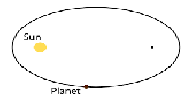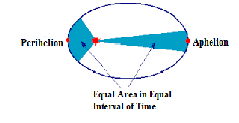# State and explain the implications of each of Kepler's three laws of planetary motion.

## Question:

State and explain the implications of each of Kepler's three laws of planetary motion.

## Kepler's Law of Planetary Motion

Johannes Kepler was an astronomer who established three laws that govern the motion of planets around the sun. The same law is applicable to the motion of satellites around the planets.

Kepler's first law: Law of Orbits: All planets move in an elliptical orbit around the sun, with the sun at one of its foci.

This law states that the path of a planet around the sun is an ellipse and not a circle. An ellipse has two foci, the sun is at one of the two foci of the elliptical path.Kepler's second law: Law of Area: Each planet revolves around the sun in such a way that the line joining the planet to the sun sweeps over equal areas in equal intervals of time.

Since the line joining the planet and the sun sweeps over an equal area in equal intervals of time, it means that a planet moves faster when it is closer to the sun and moves slowly when it is farther from the sun.Kepler's third law:cLaw of Periods: The square of the period of revolution of any planet around the sun is directly proportional to the cube of its mean distance from the sun.

If the period of a planet around the sun is T and the mean radius of the orbit is r, then

{eq}T^{2} \propto r^{3} {/eq}

{eq}T^{2} = k r^{3} {/eq}

where k is a constant of proportionality. So, larger the distance of a planet from the sun larger will be its time of revolution around the sun.